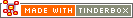== (equality)Operator Type: Mathematical Operator Scope of Action: Item

Post v4.6, the operator to test equality (i.e. 'is the same as') is two equals signs '=='. This replaces older syntax where a single equals sign was used contextually for both assignment and equality tests.

This operator is used either in agent queries or in the conditional part of an if(condition){action} code.

Equality testing can be negated, i.e. tested for a non-match, or combined with greater/less than for a range of tests as further explored in Basic Comparison Codes.

 Up: Item-based operators Previous: = (assignment) Next: abs(argument)

[Last updated: 14 Dec 2009, using v5.0]